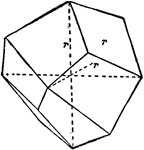### Deltohedron

Principal forms of the isometric system: deltohedron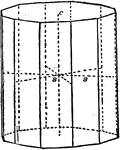### Dihexagonal Prism

Principal forms of the hexagonal system: dihexagonal prism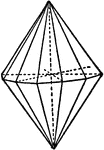### Dihexagonal Pyramid

Principal forms of the hexagonal system: dihexagonal pyramid.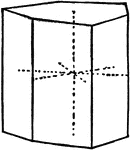### Ditrigonal Prism

Principal forms of the hexagonal system: ditrigonal prism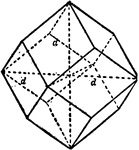### Dodecahedron

Principal forms of the isometric system: dodecahedron.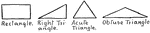### Forms

Showing different types of forms or shapes: rectangle, right triangle, acute triangle, and obtuse triangle.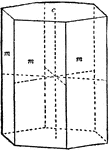### Hexagonal Prism First Order

Principal forms of the hexagonal system: hexagonal prism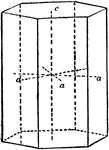### Hexagonal Prism Second Order

Principal forms of the hexagonal system: hexagonal prism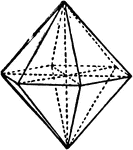### Hexagonal Pyramid First Order

Principal forms of the hexagonal system: hexagonal pyramid.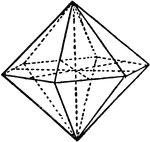### Hexagonal Pyramid Second Order

Principal forms of the hexagonal system: hexagonal pyramid.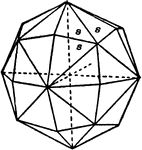### Hexoctahedron

Principal forms of the isometric system: hexoctahedron.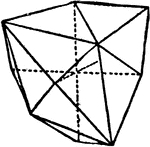### Hextetrahedron

Principal forms of the isometric system: hextetrahedron.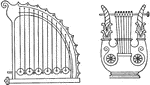### Lyre

"Two forms of the old Greek lyre." -Foster, 1921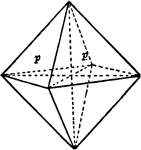### Octahedron

Principal forms of the isometric system: octahedron.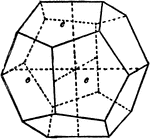### Pyritohedron

Principal forms of the isometric system: pyritohedron.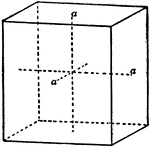### Rectangular Prism

Principal forms of the isometric system: rectangular prism.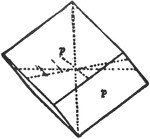### Rhombohedron

Principal forms of the hexagonal system: rhomohedron.### Scalenohedron

Principal forms of the hexagonal system: scalenohedron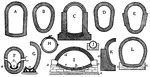### Collection of Sewer Cross-Sections

"A conduit or canal constructed, especially in a town or city, to carry off superfluous, water, soil,…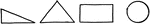### Shapes

Four different types of forms or shapes: right triangle, isosceles triangle, rectangle, and circle.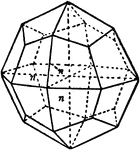### Tetragonal Trisoctahedron

Principal forms of the isometric system: tetragonal trisoctahedron.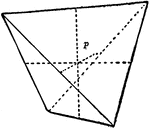### Tetrahedron

Principal forms of the isometric system: tetrahedron.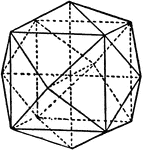### Tetrahexahedron

Principal forms of the isometric system: tetrahexahedron.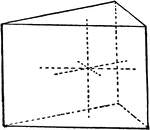### Trigonal Prism

Principal forms of the hexagonal system: trigonal prism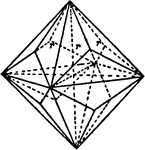### Trigonal Trisoctahedron

Principal forms of the isometric system: trigonal trisoctahedron.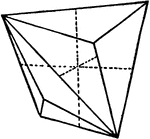### Tristetrahedron

Principal forms of the isometric system: tristetrahedron.